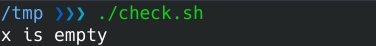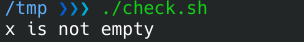Check if a Variable Is Empty in Bash

This article shows how to check if a variable is empty in Linux Bash. Then, we will examine different methods that check the length or content of the value.

There is more than one way to check if a variable is empty in Bash. We can check if the length of the value is zero or if its content is empty.

Check if a Variable Is Empty in Bash - With the -z Flag

The -z flag checks if the length of the variable is zero. Returns true if it is zero.

if [[ -z "\$x" ]]; then
echo "x is empty"
else
echo "x is not empty"
fiCheck if a Variable Is Empty in Bash - With the -n Flag

The -n flag checks if the length of the variable is nonzero. Returns false if it is zero.

x="Non-empty variable"
if [ -n "\$x" ]; then
echo "x is not empty"
else
echo "x is empty"
fiCheck if a Variable Is Empty in Bash - Compare With Empty String

We can check if the value is empty by comparing it with "".

x="Non-empty variable"
if [[ "\$x" == "" ]]; then
echo "x is empty"
else
echo "x is not empty"
fiCheck if a Variable Is Empty in Bash - Check With Replace Method

If x is defined, the expression is replaced with test, otherwise null.

if [ \${x:+test} ]; then
echo "x is not empty"
else
echo "x is empty"
fiWrite for us
DelftStack articles are written by software geeks like you. If you also would like to contribute to DelftStack by writing paid articles, you can check the write for us page.

Related Article - Bash Variable

• Assign Default Value in Bash
• Check if Variable Is Empty in Bash